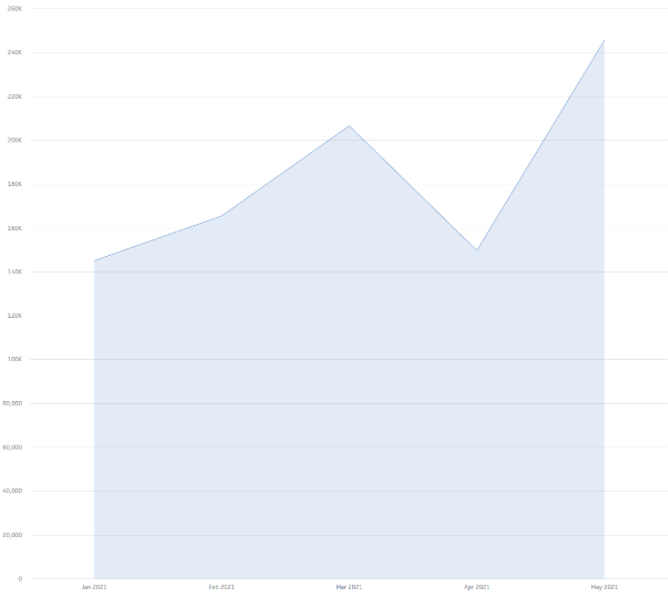In PowerMetrics, you can select one of three aggregation methods for your data: Transactional Values, Current Values, or Periodic Summary. The method you choose will determine how your metric displays data points over time. Here are some examples of metrics using these different aggregation methods!

Transactional values: Select this option if you want to include every value for a time period to create the total of values. For example, you may have a spreadsheet that includes all sales transactions for the month of January. In this case, you want to sum all values to get the total values for the month.

Example: the data below is for individual transactions on an online store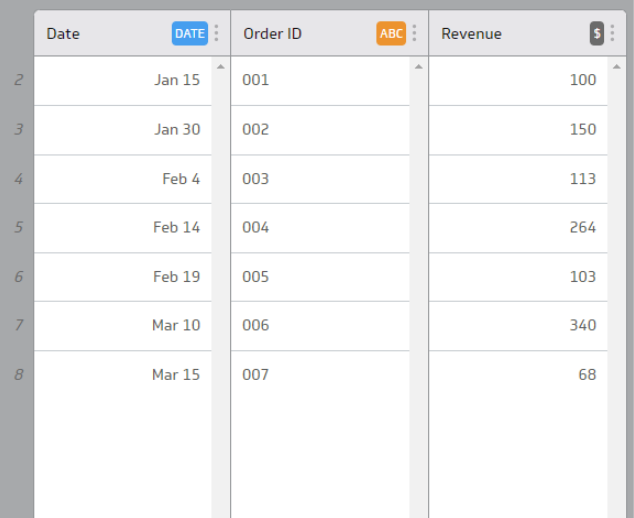Since each of these rows is itself an individual transaction, where all orders add up to total revenue, this data is best suited for Transactional Values. Transactional data can often be found in Quickbooks, Stripe, and Shopify data.

Here is this Transactional Values data as a Waterfall Chart: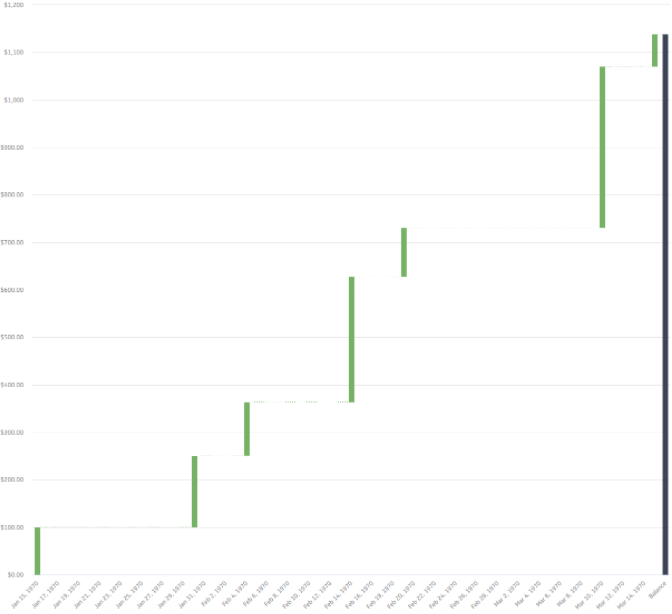Note how each individual transaction is represented in this chart and add up to a total revenue quantity.

Current Values: Select this option if your data includes current value totals for specific points in time. For example, if you are tracking social media followers, each import gives you the current value, which is the total of all values for the time period.

Example: the data below is an ongoing total of social media followers: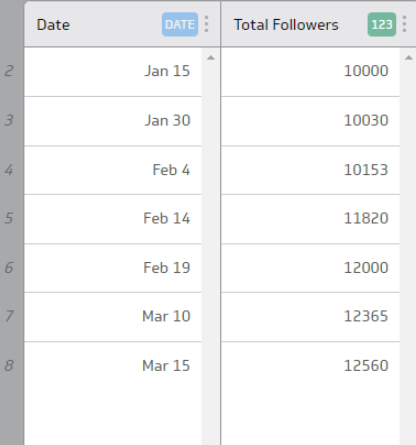Since this data is tracking total followers for each date, not new follower gains, this data is best suited for Current Values. This ensures that the total current amount of followers displayed on your metric is the most recent followers total, rather than a sum of all the listed follower counts. Current value data is often found in Twitter, LinkedIn, and Instagram data.

Here is this Current Values data as a line chart by month: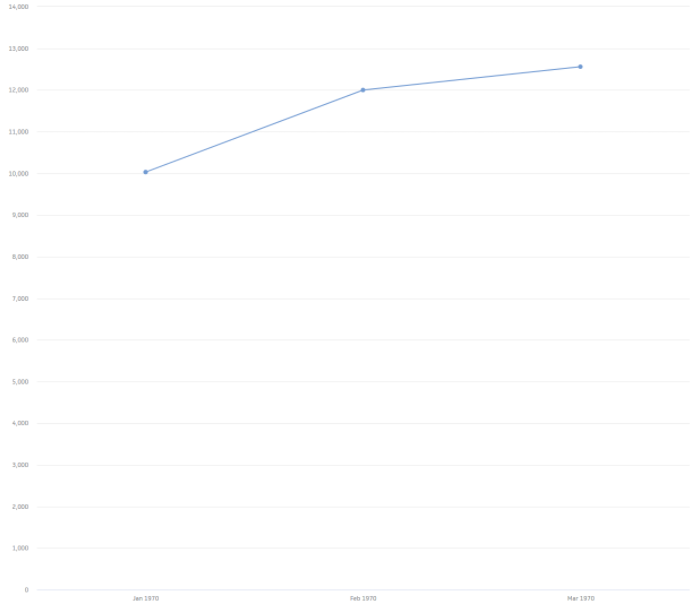Notice how only the most recent data for each month is returned when displaying the data over month

Periodic Summary: (Hour, Day, Week, Month, Year)  Select this option if each value in your data is a summary of a time period. For example, with Google Analytics "sessions by day" data, the last value of the day is a summary for that day. Your data is collected again on the following day, beginning at zero. In such cases, the last value is the complete summary of the day. To calculate the metric's value for a month, you sum the last value for each day in the month. When you select this option, you also select a time period from the drop-down list below it (Hour, Day, Week, Month, or Year).

Example: the data below is a month-by-month summary of website visitors: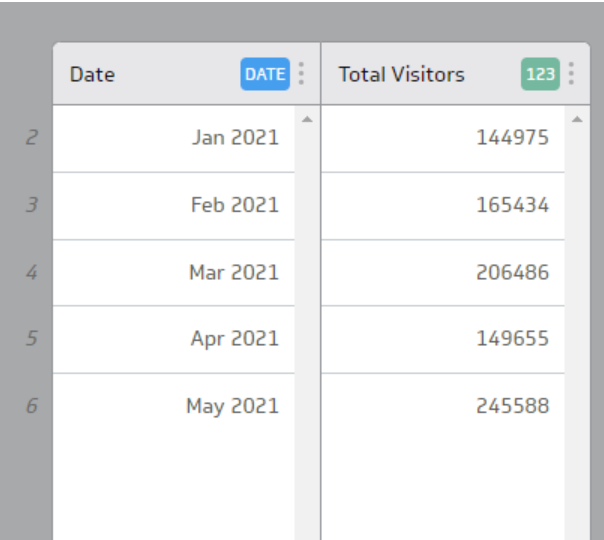Since this data is tracking the monthly total of visitors, and that total resets to 0 after each month, this data is best suited for Periodic Summary (Month). In general, if your data is separated into regular intervals (ie every Hour, Day, Week, Month, Year), with each interval representing the total value for that interval, you’ll want to select a Periodic Summary. You can often find period summary data in Google Analytics and Facebook Ads data.

Here is this Periodic Summary data in a line chart: